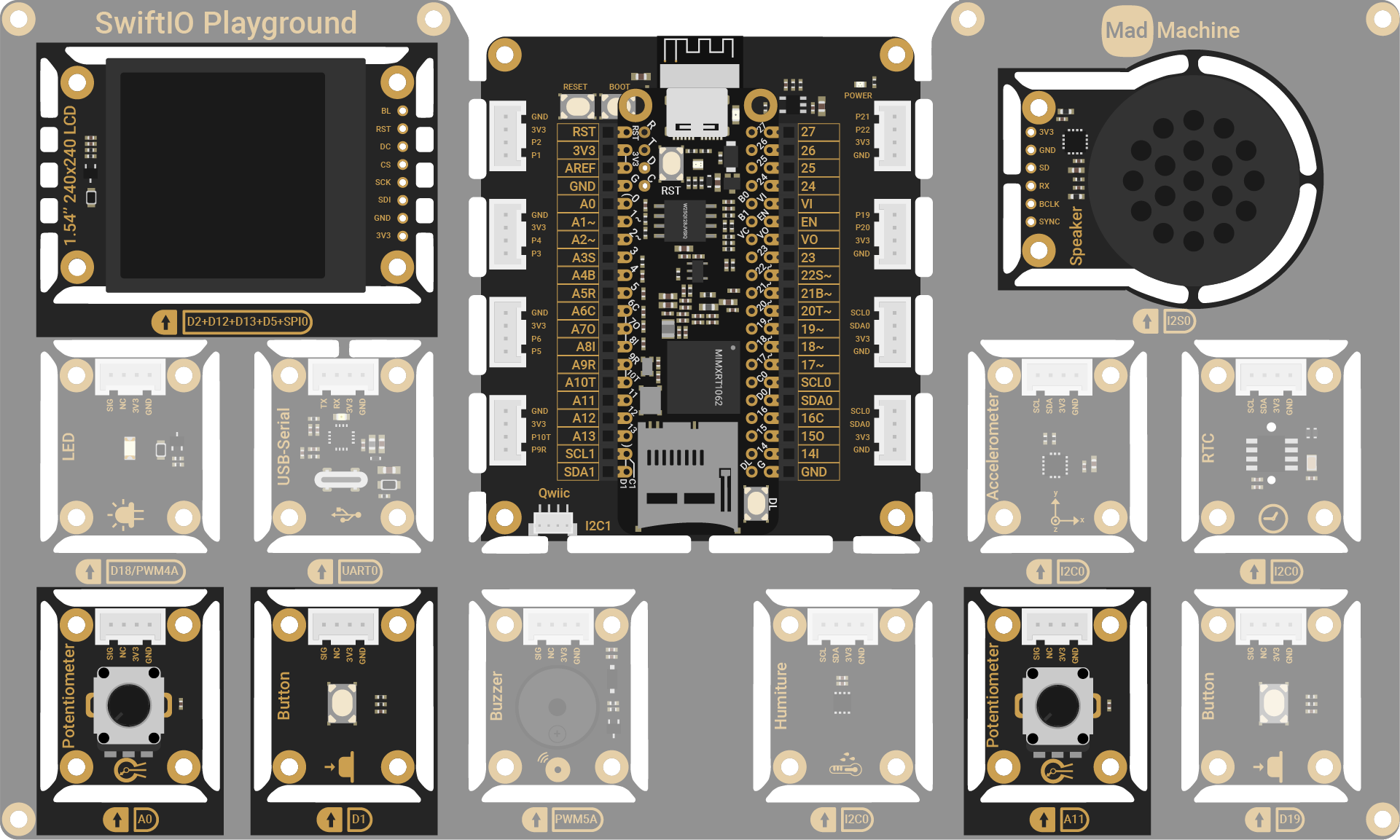# Pong

Let's play the pong game in this project. You rotate the potentiometers (A0, A11) to move two paddles in order to hit the ball back and forth.

## Circuit​

The two potentiometers (A0, A11) are used to move paddles. The button (D1) is used to reset the game. The speaker (I2S0) plays sound effects during the game.## Project overview​

• The game will start with the ball moving at a specified speed from its starting position. The ball will move in both the x and y directions.
• Each player rotates a potentiometer to move the paddle in order to hit the ball.
• Handle collision:
• Wall Collision: If the ball collides with the top or bottom wall, it will bounce off and change its vertical direction.
• Paddle Collision: If the ball collides with a paddle, it will bounce off and change its horizontal direction.
• Scoring:
• If a player fails to hit the ball and it passes the paddle, the opponent will score a point. The ball's position and speed will be reset for the next serve.
• If either player reaches the score limit, the game ends.
• The players can press the reset button to start a new game with scores reset to zero.

## Example code​

``// Import the SwiftIO to control all the pins.import SwiftIO// Import MadBoard to use the pin ids.import MadBoard// Import ST7789 driver to communicate with the screen.import ST7789@mainpublic struct Pong {    public static func main() {        let speaker = I2S(Id.I2S0, rate: 44_100)        // Initialize the SPI pin.        let spi = SPI(Id.SPI0, speed: 30_000_000)                // Initialize the pins used for the screen.        let bl = DigitalOut(Id.D2)        let rst = DigitalOut(Id.D12)        let dc = DigitalOut(Id.D13)        let cs = DigitalOut(Id.D5)        // Initialize the LCD using the pins above.         // Rotate the screen to keep the original at the upper left.        let screen = ST7789(spi: spi, cs: cs, dc: dc, rst: rst, bl: bl, rotation: .angle90)        // Initialize the analog pins for the potentiometers.        let leftPot = AnalogIn(Id.A0)        let rightPot = AnalogIn(Id.A11)        // Initialize the pin for the button used to reset the game.        let resetButton = DigitalIn(Id.D1)        // Start the game.        var game = PongGame(leftPot: leftPot, rightPot: rightPot, screen: screen, speaker: speaker)        var lastButtonState = false        while true {            /// Check if the reset button is pressed.            if resetButton.read() {                lastButtonState = true            }            /// Reset the game after the button is released.            if !resetButton.read() && lastButtonState {                game.reset()                lastButtonState = false            }            // Play the game.             // Rotate the potentiometers to move two paddles on the screen to hit the ball.            // If the ball hits the wall, the opposite player scores.            game.play()            sleep(ms: 10)        }    }}``